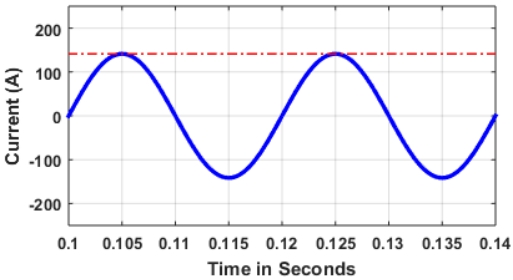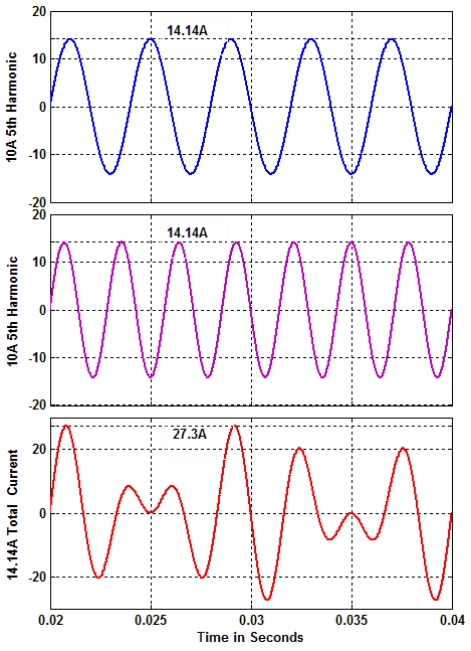# Knowledge Center

#### Importance of True RMS Harmonic Current Rating in Active Filtering Applications

RMS stands for Root Mean Square and it is equivalent representation of AC current value to DC current that would result in same active power consumption in a resistive load. A pure sinusoidal 100A RMS current waveform is shown below:

For pure sinusoidal system, the peak value of current/voltage is expressed as: $$Peak \ value ( \ sinusoidal \ current) = \sqrt{2} \times RMS \ value = 1.414 \times RMS \ value$$ Thus, 100A RMS sinusoidal current will have 141.4A peak current.

On the contrarily, the harmonic current can have peak current up to 2.2 times the RMS value, that is, $$Peak \ value ( \ harmonic \ current) = 2.2 \times RMS \ value$$The below harmonic current is 100A RMS, however its peak current value is above 200A and not 141.4A as it was case for pure sinusoidal case.

Therefore, it is very important that the rating of Active Harmonic Filters (AHFs) is determined based on true harmonic RMS current that can compensate 2.2 times peak current and not 1.414 times. If AHFs are rated based on 1.414 factor, for highly distorted harmonic currents, there would be significant derating, that is, the unit will not be able to provide the desired full compensation. This is illustrated with the following example:

Let us consider a harmonic current composed of 5th and 7th harmonics with each having 10A RMS current value. The profiles of these 5th and 7th harmonic currents along with the total current are shown below.

One can notice that the individual harmonic currents have peaks of 1.414 times RMS. However, the net current has peak value of 27.3A.

#### Total current:

$$\ Net \ RMS \ harmonic \ current \ value = \sqrt{10^2 + 10^2} = 14.14A$$

$$\ Peak \ current \ value ( \ for \ sinusoidal) = 14.14A \times \sqrt{2} = 20A$$

Actual peak harmonic current value = 27.3A [>1.93 times RMS Value; in this case]### Impact on AHF compensation and Derating:

Assume a 15A AHF is selected to compensate the above harmonic current with following continuous peak current ratings:

1. 1.414 times RMS value
2. $$\ Peak \ harmonic \ compensation = 15 \times \sqrt{2} = 21.21A$$

$$\ AHF \ RMS \ output \ current = \ AHF \ Rating \times {\ Peak \ AHF \ Current \over \ Peak \ Load \ Current} = 15 \times { 21.21\over 27.3 } = 11.65A$$

$$\ Derating = ({ 21.21 \over 27.3 } -1 ) \times 100 = 22.3 \%$$

AHF output current = 77.7% out of rated 100% (partial compensation only)

3. 2.2 times RMS value
4. $$\ Peak \ compensation = 15 \times \ 2.2 = 33A$$

$$\ Derating = \ Not \ Required$$

$$\ RMS \ Current \ output = 100 \%$$

InstaSine AHFs are designed for 2.2 times peak current value and thus, our AHF units assure that there is no derating even when the load currents are highly distorted.